T Relativistic Complex|crimson publishers.com
• Submissions# Relativistic Complex

Boris Dzhechko*

City of Sterlitamak, Bashkortostan, Russia

*Corresponding author: Boris Dzhechko, City of Sterlitamak, Bashkortostan, Russia

Submission: November 19, 2018;Published: November 26, 2018Volume1 Issue3
November 2018

#### Opinion

In the thought experiments of the theory of relativity there is always a movement of the signal at the speed of light. In this case, the light signal in this theory exists only in the imagination. However, in new Cartesian physics, it exists in reality as a shift of space propagating with the speed of light, because according to the principle of identity of physical space and matter of Descartes, the real world is created by a single moving substance, which should be called space-matter, there is nothing else in it. The beginning shift of space ends on itself with filling with the speed of light of emptiness formed behind it as a result of its shift. In addition, space serves as a conductor of electromagnetic waves, representing its wave-like shifts.

Thus, each corpuscle, which is a stationary state of space-matter, moving at a speed of v, creates a wave of shift of space moving at the speed of light c, and the ratio v/c becomes an argument in formulas that take into account the speed limit of its movement by the speed of light. In addition, this ratio in atomic physics characterizes the electron in the orbit of Bohr and called the fine structure constant, which allows including this constant in the Lorentz transformation formula. For example,In here:the speed of movement of electrons on the first orbital of Bohr; ch - the pressure of the universe, expressed the as flow forces through a closed surface.

The appearance of the Planck constant in the Lorentz transformation formula indicates that quantum mechanics and relativity are compatible, if we accept the statement of new Cartesian physics that the movement of space relative to itself can only occur circularly or oscillatory way. The rectilinear motion of space, she believes, is a movement along the trajectory of an infinitely large radius of curvature or on an infinitely small segment of the trajectory of motion. For this reason, the Planck constant as the moment of momentum is the value characterizing the motion in a circular orbit. In this case, it can be assumed that the relativistic increase in body mass occurs as a result of the accumulation of energy in the wave of shift, the higher the speed of motion of a body, the more powerful of wave of shift of space-matter.

Dividing the expression to 2π, we get:The transition in this formula from the length of the circle to the radius is natural and necessary, since the Planck constant contained in it is the moment of the force pulse, the formula of which contains the radius, and not the length of the circle. A rotating coordinate system is not inertial, but a circle is defined as the perimeter of a polygon that has an infinite number of sides that become infinitesimal arcs, and the direct movement along them is inertial, and as a result you can getWhere: R0 is the radius of the trajectory in its own frame of reference; R is the radius of the trajectory in a moving frame of reference. We obtained the function of the orbital velocity depending on two interdependent radii - R⋜R0 characterizing the space shift. Substituting this speed value in the formula of the centrifugal force,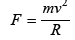we obtain the formula of the holding force during rotation, which should be called the law of universal gravitation of Lorentz: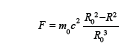Its uniqueness lies in the fact that it contains energy equivalent to the rest mass, and the ratiocharacterizes the share of shear energy in it.

Thus, if we reduce the infinitely large radius of curvature of the trajectory to an infinitely small value, we obtain inertial reference systems moving rectilinearly on infinitely small segments of the path and relativistic formulas for the rotation of the space inside the micro particles.and inversion geometry formulas

The figure geometrically shows the ratio of quantum and relativistic physical quantities:

(Figure 1)

Figure 1:Geometric Relationship of magnitudes of big formula Lorentz transformations in corpuscle.Where:

rest mass m0;

the wavelength of de Broglie R0 = λBrog;

time in its own reference frame T0;

the speed of light c;

Planck constant h;

power flow through a closed surface ch;

electric charge of electron e;

Compton wavelength λCom;

gravitational constant γ;

gravitational mass M in a moving frame of reference.

Consider the radii Rand R0= λBrog on the complex plane z = x + yi as modules of complex expression Z = X + Yi . So we can write a complex function from the real variable, reflecting the found space relations on the set of complex numbers:The real part of this complex expression is the Compton wavelength, and the imaginary part is the length reduced as a result of motion. Similar complexes can be made for other physical quantities, for example:Figure 2: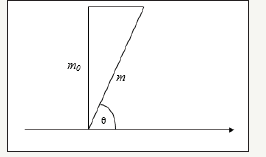For the time will have a similar:If we have a velocity field, then each point the complex function of the real variable corresponds to a relativistic complex, the real part of which will containand the imaginary part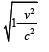The speed value at the point changes the value of the complex, but does not show its rotation. In order for it to acquire rotation, it is necessary to multiply it by a complex number with the module equal to one and the argument φ, i.e.thenMany formulas of physical laws do not limit the increase in the speed of movement by the speed of light. This is the law of conservation of momentum, Newton’s law of universal gravitation, Coulomb’s law, etc. Note that the relativistic complex admits the excess of the speed of motion of the space of the speed of light, since at v≥c, it will be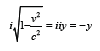Thus, mathematically formalized approval new Cartesian physics that when the excess speed of movement of space speed of light, occurs the radiation of excess energy.

© 2018 Boris Dzhechko. This is an open access article distributed under the terms of the Creative Commons Attribution License , which permits unrestricted use, distribution, and build upon your work non-commercially.

﻿

We at Crimson Publishing are a group of people with a combined passion for science and research, who wants to bring to the world a unified platform where all scientific know-how is available read more...